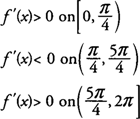## Increasing/Decreasing Functions

The derivative of a function may be used to determine whether the function is increasing or decreasing on any intervals in its domain. If f′(x) > 0 at each point in an interval I, then the function is said to be increasing on I. f′(x) < 0 at each point in an interval I, then the function is said to be decreasing on I. Because the derivative is zero or does not exist only at critical points of the function, it must be positive or negative at all other points where the function exists.

In determining intervals where a function is increasing or decreasing, you first find domain values where all critical points will occur; then, test all intervals in the domain of the function to the left and to the right of these values to determine if the derivative is positive or negative. If f′(x) > 0, then f is increasing on the interval, and if f′(x) < 0, then f is decreasing on the interval. This and other information may be used to show a reasonably accurate sketch of the graph of the function.

Example 1: For f(x) = x 4 − 8 x 2 determine all intervals where f is increasing or decreasing.

The domain of f(x) is all real numbers, and its critical points occur at x = −2, 0, and 2. Testing all intervals to the left and right of these values for f′(x) = 4 x 3 − 16 x, you find thathence, f is increasing on (−2,0) and (2,+ ∞) and decreasing on (−∞, −2) and (0,2).

Example 2: For f(x) = sin x + cos x on [0,2π], determine all intervals where f is increasing or decreasing.

The domain of f(x) is restricted to the closed interval [0,2π], and its critical points occur at π/4 and 5π/4. Testing all intervals to the left and right of these values for f′(x) = cos x − sin x, you find thathence, f is increasing on [0, π/4](5π/4, 2π) and decreasing on (π/4, 5π/4).